JP5101675B2 - Supply-demand balance control device - Google Patents

Info

Publication number
JP5101675B2
JP5101675B2 JP2010201987A JP2010201987A JP5101675B2 JP 5101675 B2 JP5101675 B2 JP 5101675B2 JP 2010201987 A JP2010201987 A JP 2010201987A JP 2010201987 A JP2010201987 A JP 2010201987A JP 5101675 B2 JP5101675 B2 JP 5101675B2
Authority
JP
Japan
Prior art keywords
demand
power
supply
amount
unit
Prior art date
Legal status (The legal status is an assumption and is not a legal conclusion. Google has not performed a legal analysis and makes no representation as to the accuracy of the status listed.)
Active
Application number
JP2010201987A
Other languages
Japanese (ja)
Other versions
JP2012060789A (en
Inventor

Original Assignee

Priority date (The priority date is an assumption and is not a legal conclusion. Google has not performed a legal analysis and makes no representation as to the accuracy of the date listed.)
Filing date
Publication date
Application filed by 株式会社東芝 filed Critical 株式会社東芝
Priority to JP2010201987A priority Critical patent/JP5101675B2/en
Publication of JP2012060789A publication Critical patent/JP2012060789A/en
Application granted granted Critical
Publication of JP5101675B2 publication Critical patent/JP5101675B2/en
Application status is Active legal-status Critical
Anticipated expiration legal-status Critical

• 230000001276 controlling effects Effects 0 abstract 1

Images

•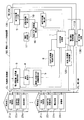•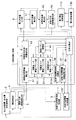•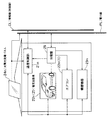•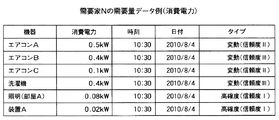•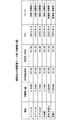••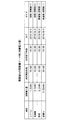•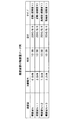•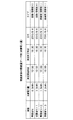•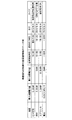••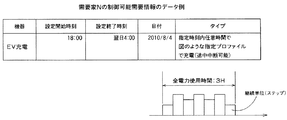•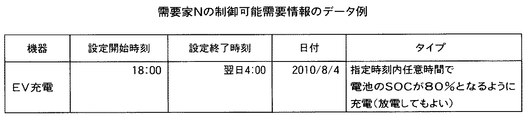••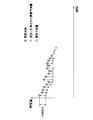•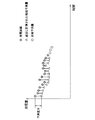•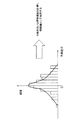•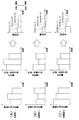•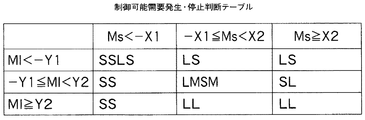•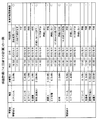•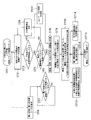•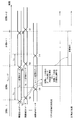••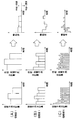•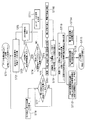•Classifications

• HELECTRICITY
• H02GENERATION; CONVERSION OR DISTRIBUTION OF ELECTRIC POWER
• H02JCIRCUIT ARRANGEMENTS OR SYSTEMS FOR SUPPLYING OR DISTRIBUTING ELECTRIC POWER; SYSTEMS FOR STORING ELECTRIC ENERGY
• H02J3/00Circuit arrangements for ac mains or ac distribution networks
• H02J3/12Circuit arrangements for ac mains or ac distribution networks for adjusting voltage in ac networks by changing a characteristic of the network load
• H02J3/14Circuit arrangements for ac mains or ac distribution networks for adjusting voltage in ac networks by changing a characteristic of the network load by switching loads on to, or off from, network, e.g. progressively balanced loading
• HELECTRICITY
• H02GENERATION; CONVERSION OR DISTRIBUTION OF ELECTRIC POWER
• H02JCIRCUIT ARRANGEMENTS OR SYSTEMS FOR SUPPLYING OR DISTRIBUTING ELECTRIC POWER; SYSTEMS FOR STORING ELECTRIC ENERGY
• H02J3/00Circuit arrangements for ac mains or ac distribution networks
• H02J2003/003Load forecast, e.g. method and systems for forecasting future load demand
• HELECTRICITY
• H02GENERATION; CONVERSION OR DISTRIBUTION OF ELECTRIC POWER
• H02JCIRCUIT ARRANGEMENTS OR SYSTEMS FOR SUPPLYING OR DISTRIBUTING ELECTRIC POWER; SYSTEMS FOR STORING ELECTRIC ENERGY
• H02J3/00Circuit arrangements for ac mains or ac distribution networks
• H02J2003/007Simulating, e. g. planning, reliability check, modeling
• YGENERAL TAGGING OF NEW TECHNOLOGICAL DEVELOPMENTS; GENERAL TAGGING OF CROSS-SECTIONAL TECHNOLOGIES SPANNING OVER SEVERAL SECTIONS OF THE IPC; TECHNICAL SUBJECTS COVERED BY FORMER USPC CROSS-REFERENCE ART COLLECTIONS [XRACs] AND DIGESTS
• Y02TECHNOLOGIES OR APPLICATIONS FOR MITIGATION OR ADAPTATION AGAINST CLIMATE CHANGE
• Y02BCLIMATE CHANGE MITIGATION TECHNOLOGIES RELATED TO BUILDINGS, e.g. HOUSING, HOUSE APPLIANCES OR RELATED END-USER APPLICATIONS
• Y02B70/00Technologies for an efficient end-user side electric power management and consumption
• Y02B70/30Systems integrating technologies related to power network operation and communication or information technologies for improving the carbon footprint of the management of residential or tertiary loads, i.e. smart grids as climate change mitigation technology in the buildings sector, including also the last stages of power distribution and the control, monitoring or operating management systems at local level
• Y02B70/32End-user application control systems
• Y02B70/3208End-user application control systems characterised by the aim of the control
• Y02B70/3225Demand response systems, e.g. load shedding, peak shaving
• YGENERAL TAGGING OF NEW TECHNOLOGICAL DEVELOPMENTS; GENERAL TAGGING OF CROSS-SECTIONAL TECHNOLOGIES SPANNING OVER SEVERAL SECTIONS OF THE IPC; TECHNICAL SUBJECTS COVERED BY FORMER USPC CROSS-REFERENCE ART COLLECTIONS [XRACs] AND DIGESTS
• Y02TECHNOLOGIES OR APPLICATIONS FOR MITIGATION OR ADAPTATION AGAINST CLIMATE CHANGE
• Y02EREDUCTION OF GREENHOUSE GAS [GHG] EMISSIONS, RELATED TO ENERGY GENERATION, TRANSMISSION OR DISTRIBUTION
• Y02E60/00Enabling technologies or technologies with a potential or indirect contribution to GHG emissions mitigation
• Y02E60/70Systems integrating technologies related to power network operation and communication or information technologies mediating in the improvement of the carbon footprint of electrical power generation, transmission or distribution, i.e. smart grids as enabling technology in the energy generation sector
• Y02E60/76Computer aided design [CAD]; Simulation; Modelling
• YGENERAL TAGGING OF NEW TECHNOLOGICAL DEVELOPMENTS; GENERAL TAGGING OF CROSS-SECTIONAL TECHNOLOGIES SPANNING OVER SEVERAL SECTIONS OF THE IPC; TECHNICAL SUBJECTS COVERED BY FORMER USPC CROSS-REFERENCE ART COLLECTIONS [XRACs] AND DIGESTS
• Y04INFORMATION OR COMMUNICATION TECHNOLOGIES HAVING AN IMPACT ON OTHER TECHNOLOGY AREAS
• Y04SSYSTEMS INTEGRATING TECHNOLOGIES RELATED TO POWER NETWORK OPERATION, COMMUNICATION OR INFORMATION TECHNOLOGIES FOR IMPROVING THE ELECTRICAL POWER GENERATION, TRANSMISSION, DISTRIBUTION, MANAGEMENT OR USAGE, i.e. SMART GRIDS
• Y04S20/00Systems supporting the management or operation of end-user stationary applications, including also the last stages of power distribution and the control, monitoring or operating management systems at local level
• Y04S20/20End-user application control systems
• Y04S20/22End-user application control systems characterised by the aim of the control
• Y04S20/222Demand response systems, e.g. load shedding, peak shaving
• YGENERAL TAGGING OF NEW TECHNOLOGICAL DEVELOPMENTS; GENERAL TAGGING OF CROSS-SECTIONAL TECHNOLOGIES SPANNING OVER SEVERAL SECTIONS OF THE IPC; TECHNICAL SUBJECTS COVERED BY FORMER USPC CROSS-REFERENCE ART COLLECTIONS [XRACs] AND DIGESTS
• Y04INFORMATION OR COMMUNICATION TECHNOLOGIES HAVING AN IMPACT ON OTHER TECHNOLOGY AREAS
• Y04SSYSTEMS INTEGRATING TECHNOLOGIES RELATED TO POWER NETWORK OPERATION, COMMUNICATION OR INFORMATION TECHNOLOGIES FOR IMPROVING THE ELECTRICAL POWER GENERATION, TRANSMISSION, DISTRIBUTION, MANAGEMENT OR USAGE, i.e. SMART GRIDS
• Y04S40/00Systems for electrical power generation, transmission, distribution or end-user application management characterised by the use of communication or information technologies, or communication or information technology specific aspects supporting them
• Y04S40/20Information technology specific aspects
• Y04S40/22Computer aided design [CAD]; Simulation; Modelling

Abstract

A first predicting unit predicts a power demand required by each customer. A first reliability value calculator calculates the reliability value of the predictive power demand for each customer. Predicted power supply from a power system is calculated with respect to each power generating unit. A second reliability value calculator calculates the reliability value of the predictive power supply for each power generating unit. A balancing unit obtains a supply-demand margin level based on the difference between: a first weighted sum obtained by performing weighted sum on the predictive power demand and a weight depending on the reliability value; and a second weighted sum obtained by performing weighted sum on the predictive power supply and a weight depending on the reliability value. A plan creator creates a plan for controlling power used by the controllable appliance of the customer based on the supply-demand margin level.

Description

An embodiment of the present invention relates to a supply and demand balance control device in a smart grid, for example.

If the difference between demand power and purchased power, which could not be predicted at the planning stage, is detected and power quality cannot be maintained, it is possible to connect with other power system supply and demand control devices to which the power is received at a minimum cost. An accommodation determination device that determines the amount of power received in real time is known.

Each consumer is to conclude a power demand contract of a predetermined value, and whether to exceed the contracted power amount is determined by the accommodation determination device.

The power demand contract has a difference from the actually required power demand, and it is necessary to expect the power demand that is not actually consumed in the contract. Therefore, in this accommodation determination apparatus, there is a problem that a plan for inadvertently generating a large amount of power is created. When excessive power generation is performed, a large-scale power storage device that stores this surplus power is required.

In particular, when electric power with uncertain power generation, such as solar power generation or wind power generation, is supplied, there is a possibility that a predetermined contracted power amount cannot be supplied depending on the weather and weather conditions. The versatility determination device functioning in real time has a problem of increasing the possibility of easily generating an additional power request. If surplus addition is performed, a large-scale power storage device for storing surplus power is required.

As another prior art, the following is known. In this prior art, each power generation output target value is generated from the total power generation output by the load distribution calculation method. At this time, based on the expected value that is the predicted value of the power generation output and the variance that represents the reliability of the expected value, if the reliability of the predicted value is high, the economic efficiency that minimizes the fuel cost by economic load distribution Calculate a high power output target. On the other hand, when the reliability of the predicted value is low, the power generation reserve target of the distributed power source is secured and the power generation output target having high responsiveness is calculated.

In this prior art, uncertainties of solar power generation and wind power generation are expressed as reliability, and the assigned load distribution is changed according to the reliability. At this time, the uncertainty of the demand itself is not taken into consideration.

For this reason, like the prior art described above, power that is not actually consumed is generated, and as a result, there is a possibility that excessive power generation is performed. In addition, there is a problem that a large-scale power storage device that absorbs surplus power generated at this time must be built and held in the system.

JP 2000-333369 A JP 2008-271723 A

The present invention provides a supply and demand balance control device that realizes supply and demand control that does not require a large-scale power storage device by appropriately balancing demand and supply.

As one aspect of the present invention, there is provided a supply and demand balance control device that makes a supply plan for a predetermined period.

The supply and demand balance control device includes a collection control unit, a first storage unit, a first prediction unit, a second storage unit, a first reliability value calculation unit, a power reception unit, a distribution unit, and a power supply amount. An acquisition unit, a third storage unit, a second prediction unit, a fourth storage unit, a second confidence value calculation unit, a balance adjustment unit, and a planning unit.

The collection control unit collects, from the consumer, power demand data, which is the amount of power consumed by a demand device including a controllable demand device that can be controlled from the outside and that is disposed in the consumer. To do.

The first storage unit stores a history of the power demand for each consumer.

The first prediction unit calculates a power demand prediction amount required for each consumer in the predetermined period based on a history of the power demand amount in the first storage unit.

The second storage unit stores the predicted power demand calculated by the prediction unit.

The first reliability value calculation unit includes the past power demand amount in the first storage unit and the past power demand prediction amount calculated by the first prediction unit with respect to the past power demand amount. Based on the difference, a confidence value for the predicted power demand calculated by the first prediction unit is calculated for each consumer.

The power receiving unit receives supply of power from a power system that generates power by a plurality of power generation means.

The distribution unit distributes the power supplied to the power receiving unit to the consumers.

The power supply amount acquisition unit acquires data of the power supply amount supplied from the power system from the power system for each power generation means.

The third storage unit stores a history of power supply amount for each power generation means.

The second prediction unit calculates a predicted power supply amount that is predicted to be supplied in the predetermined period for each power generation unit, based on the history stored in the third storage unit.

The fourth storage unit stores the predicted power supply amount calculated by the second prediction unit.

The second reliability value calculation unit includes the past power supply amount in the third storage unit, and the past power supply prediction amount calculated by the second prediction unit with respect to the past power supply amount. Based on the deviation amount, a reliability value for the predicted power supply amount predicted by the second prediction unit is calculated for each power generation means.

The balance adjustment unit is a first weighted sum obtained by weighting and summing the power demand prediction amount calculated by the first prediction unit based on a weight corresponding to the reliability value calculated by the first confidence value calculation unit, Based on the difference between the power supply prediction amount calculated by the second prediction unit and the second weighted sum obtained by weighting the weight according to the reliability value calculated by the second reliability value calculation unit, the supply and demand margin Ask for.

The planning unit plans power usage control of the controllable demand device in the consumer in the predetermined period based on the supply and demand margin.

1 shows a block configuration of a supply and demand control system including a supply and demand balance control device according to a first embodiment. FIG. 2 shows a block configuration of a supply planning unit in the supply-demand balance control apparatus of FIG. The example of a specific apparatus structure in a consumer is shown. The 1st example of demand amount data is shown. The 2nd example of demand amount data is shown. The 3rd example of demand amount data is shown. The 4th example of demand amount data is shown. The 5th example of demand amount data is shown. The 6th example of demand amount data is shown. The 1st example of controllable demand information is shown. The 2nd example of controllable demand information is shown. The 3rd example of controllable demand information is shown. The 4th example of controllable demand information is shown. The 5th example of controllable demand information is shown. A state in which a demand amount is predicted is schematically shown. A state of predicting the power generation amount is schematically shown. The calculation method of the confidence value of the demand forecast amount is shown. The operation of the supply-demand balance unit is shown. An example of a controllable demand occurrence / stop determination table is shown. An example of a planned supply plan is shown. The flow of operation | movement by the supply-and-demand balance control apparatus shown in FIG. 1 is shown. This shows how data is collected at sampling intervals for each consumer. An example of changing the sampling interval according to the reliability according to the second embodiment will be described. The operation | movement of the supply-and-demand balance part in 2nd Embodiment is shown. The flow of the whole operation | movement by the supply-and-demand balance control apparatus which concerns on 3rd Embodiment is shown. The example of calculation of the demand-and-demand difference which concerns on 3rd Embodiment is shown.

Hereinafter, embodiments of the present invention will be described with reference to the drawings.

(First embodiment)
FIG. 1 shows a block configuration of a supply and demand control system including a supply and demand balance control apparatus according to the first embodiment. FIG. 2 shows a block configuration of the supply planning unit 1 in the supply-demand balance control apparatus 50 of FIG.

This supply and demand balance control apparatus appropriately controls the power consumption of controllable demand devices in each consumer by appropriately predicting the power demand of a plurality of consumers and the power supply from the power grid, and also a smart grid The request | requirement of the additional electric power to electric power systems, etc. and charge / discharge to an electric power storage apparatus are performed appropriately. This balances the amount of power supplied and the power demand, reduces the generation of excess power in the power system and excessive power supply from the power system, and keeps the size of the power storage device small.

The supply and demand balance control device 50 is connected to an electric power system 100 such as a smart grid, an electric power storage device 130 that stores electric power, and a plurality of consumers A to N.

The power system 100 includes a plurality of power generation means, and generates power by the plurality of power generation means. The plurality of power generation means include, for example, a number of power generation means such as nuclear power generation means, hydroelectric power generation means, thermal power generation means, wind power generation means, solar power generation means, wave power generation means, and geothermal power generation means.

The power system 100 sends power supply amount data including the power supply amount (suppliable power amount) for each power generation means to the supply and demand balance control device 50. The power supply amount data is sent in response to a request issued from the supply and demand balance control device 50 or periodically.

The supply and demand balance control device 50 receives the power supply amount data at the power supply amount input unit (power supply amount acquisition unit, addition request unit) 9. The power supply amount data includes a label for identifying the power generation means and a schedule for the amount of power supplied by the power generation means.

There are various types of schedules. It may be power for a future time period, power supplied at the present time, or power supplied within a predetermined period from the current time. The predetermined time period may be a format in which a specific time period such as XX hours XX minutes to XX hours XX minutes is specified, or a format such as 15 minutes from XX hours XX minutes.

The power system 100 sends the generated power to a power supply and demand control device (electricity receiving unit, distribution unit) 110 via a transmission line (not shown). The power supply / demand control device 110 supplies the electric power to consumers via the power line PL, or charges the electric power storage device 130 with the electric power as necessary. In addition, the power supply / demand control apparatus 110 takes out electric power from the power storage apparatus 130 by discharging as necessary and supplies the electric power to consumers.

The consumers A to N are connected to the supply and demand balance control device 50 via an information line (control line) CL and a power line PL. The information line CL is for communicating data or a control signal between the consumers A to N and the supply and demand balance control device 50. The power line PL is for performing power transmission from the power supply / demand control apparatus 100 in the supply / demand balance control apparatus 50 to the consumers A to N.

In the consumers A to N, communication control units 21a to 21n are provided. In addition, in consumers A to N, demand devices 22a to 22n and controllable demand devices 23a to 23n are provided as power consuming devices.

The controllable demand device is a device capable of adjusting (controlling) its operation (power consumption) from the outside. The amount of power that can be controlled in this way is referred to as a controllable demand amount.

Some or all of consumers are provided with a generator such as a solar power generation panel. In the example of FIG. 1, a generator 24n is provided in the customer N. A number of other customers are also equipped with generators.

The communication control units 21a to 21n communicate with the demand devices 21a to 21n and the controllable demand devices 23a to 23n to control these devices. Further, the communication control units 21a to 21n read out and transmit the detailed information of the demand devices 21a to 21n and the controllable demand devices 23a to 23n in response to a request from the supply and demand balance control device. The detailed information on the controllable demand devices 23a to 23n includes controllable demand information (see FIGS. 10 to 14) described later.

The communication control units 21a to 21n acquire demand data including power consumption by the demand devices 21a to 21n and the controllable demand devices 23a to 23n for each device. The communication control units 21a to 21n may acquire demand data including the total power consumption consumed by the demand devices 21a to 21n and the controllable demand devices 23a to 23n. Acquisition of power consumption for each device or for the entire device can be obtained using various known methods. For example, it can be obtained using a distribution board. Moreover, the communication control part in the consumer provided with a generator acquires the data of the electric power generation amount by a generator.

The communication control units 21a to 21n receive demand data, power generation data, controllable demand information, detailed device information, and supply / demand balance control device 50 in response to a request from the supply / demand balance control device 50 or periodically. Can be sent to.

FIG. 3 shows a specific device configuration example in the customer N.

An air conditioner 22n (1) and an electric vehicle 22n (2) are provided as controllable demand devices. A photovoltaic power generation panel 24n is provided as a generator. The demand equipment 23n is not limited to a specific equipment here. The demand device 23n is a normal device that cannot be controlled from the outside (that is, the controllable demand control device 120).

In addition, a distribution board 25 is provided, and the power generated by the power system 100 or the discharge power of the power storage device 130 is received via the power supply / demand control device 110 by the power line PL. The distribution board 25 distributes the received power to the devices 22n (1), 22n (2), 23n, the communication control unit 21n, and the like that consume power in the consumer. The distribution board 25 measures the power consumption of each device, and notifies the communication control unit 21n of the measurement value (power consumption) for each device or the power consumption of the entire device.

The communication control unit 21n transmits the demand amount data including the power consumption of each device or the entire device received from the distribution board 25 to the supply and demand balance control device via the information line CL. Further, the communication control unit 21n reads detailed information of each device 22n (1), 22n (2), 23n from these devices, and transmits it to the supply-demand balance control device 50 via the information battle CL. In particular, controllable demand information of air conditioners and electric vehicles that are controllable demand devices is transmitted to the control balance control device 50. In addition, the communication control unit 21n transmits the power generation amount data by the solar power generation panel 24n to the supply and demand balance control device 50.

Hereinafter, some examples of demand amount data and controllable demand information are shown. Note that the formats of demand amount data and controllable demand information are various, and are not limited to the following examples.

4 to 9 show examples of demand amount data.

FIG. 4 shows a first example of demand data.

This example shows demand amount data in the case of acquiring power consumption at a specific time for each demand device of each consumer. In the type column, the generation accuracy and reliability of each demand are shown. This reliability is a value calculated by the demanding device in the same manner as the demand-by-reliability demand amount totaling unit 41 (see FIG. 2) described later. The occurrence accuracy is determined according to the magnitude of the reliability. In this embodiment, the reliability I is defined as high accuracy, and the reliability below II is defined as fluctuation. The meaning of reliability and occurrence accuracy will be clarified later.

FIG. 5 shows a second example of demand data.

This example shows an example of demand data when acquiring power consumption at predetermined time intervals.

FIG. 6 shows a third example of demand data. FIG. 7 shows a fourth example of demand data.

The third and fourth examples show demand power data when presenting power consumption or power consumption according to the type of consumer device.

FIG. 8 shows a fifth example of demand data. FIG. 9 shows a sixth example of demand data.

The fifth and sixth examples show demand amount data when total power consumption or total power consumption can be acquired for each consumer.

10 to 14 show data examples of controllable demand information of controllable demand devices.

FIG. 10 shows a first example of controllable demand information.

A plurality of items are defined for each consumer-controllable demand device.

The maximum power consumption is the maximum power consumption when power control is not performed, and the minimum power consumption is the minimum power consumption when power consumption is suppressed by power control. By notifying the maximum / minimum power consumption of each controllable demand device, the supply and demand balance control device 50 can accurately estimate the amount of power that can be suppressed when control is performed, as will be described later.

The setting start time and the setting end time define a controllable time zone.

The type indicates a controllable form. In this example, the EV can be controlled at any time within the specified time zone, ON / OFF can be arbitrarily controlled for lighting equipment, and the internal settings can be freely set for the air conditioner A. It can be changed.

FIG. 11 shows a second example of controllable demand information.

This example is almost the same as FIG. 10, except that the maximum power consumption and the minimum power consumption are not defined.

FIG. 12 shows a third example of controllable demand information.

This example is controllable demand information of a type that generates a specific pattern of power demand within a specified time period.

The illustrated case shows a demand for generating a specific pattern of 7 steps when charging an electric vehicle, and the start timing of each step can be arbitrarily controlled. It is sufficient that these seven steps are completed during the designated time period, and the start time of each step can be controlled according to the power supply state.

FIG. 13 shows a fourth example of controllable demand information.

This example is controllable demand information of a type in which the state of the consumer device at the time of the setting end time is specified as a condition. In the illustrated data, conditions are given so that the SOC (State Of Charge) of the battery is in a predetermined state.

FIG. 14 shows a fifth example of controllable demand information.

This example shows the control demand possible information of the type which notifies a controllable demand as a power supply adjustment margin when a consumer is a building etc. and has an original energy management system.

Within the specified time, even if the supply is zero at any time, it is possible to operate the power using the energy management system or storage battery in the building, or the case where specific power can be reduced at any time. A case is shown in which the percentage of power to be reduced can be specified.

The power demand / power generation collection control unit (hereinafter, collection control unit) 3 acquires (collects) demand data and power generation data from the consumers A to N via the information line CL. The collection control unit 3 collects controllable demand information from the consumers A to N. The collection is performed in response to a data collection request issued by the supply planning unit 1 at a predetermined interval (for example, an interval of Ts) or at a predetermined timing. In the following description, unless otherwise noted, for the sake of simplicity, it is assumed that the demand data from the consumers A to N includes the power demand for each device. Further, it is assumed that the power generation amount data from the consumer includes the power generation amount for each generator.

The power demand / power generation history storage unit (hereinafter, history storage unit) 4 stores the history of data (demand amount data, power generation amount data) collected by the collection control unit 3. That is, the history storage unit 4 also stores and holds data collected in the past. The history storage unit 4 organizes and stores demand amount data and power generation amount data collected in the past for each customer and device, and newly input demand amount data and power generation amount data. The history storage unit stores controllable demand information for each controllable demand device in the consumer. The history storage unit 4 corresponds to a first storage unit and a second storage unit.

The fluctuation demand and power generation amount prediction / reliability value calculation unit (hereinafter referred to as demand & prediction / reliability value calculation unit) 5 uses the history stored in the history storage unit 4 to determine the demand amount and generator for each consumer device for each consumer. By predicting another power generation amount, a demand prediction amount and a power generation prediction amount are obtained. In addition, the respective reliability values of the demand forecast amount and the power generation forecast amount are calculated. The demand & prediction / confidence value calculation unit (first prediction unit, first confidence value calculation unit) 5 stores the value of the demand prediction amount and the power generation prediction amount in the history storage unit 4.

The demand amount is predicted for the next section k + 1 of the current section k of the section group divided every ΔT time in the future, and further sections after that if necessary. That is, for the current time interval, the demand amount for the next interval is predicted from the demand amounts for a plurality of past time intervals. In other words, in the current section, the plan (demand / supply control) determined in the section before the present is executed in parallel. The section k + 1 corresponds to a predetermined period that is a target for planning.

The prediction method will be specifically described. In a certain time interval, a prediction model is constructed that predicts the demand amount in the next interval of the certain time interval from the demand amounts in the past plural time intervals. Then, the demand amount of the next section k + 1 is predicted from this prediction model and the demand quantities of a plurality of past time sections for the current section k. FIG. 15 schematically shows how the demand is predicted each time the section advances (the “prediction difference” described in the figure will be described later). Many models for predicting future values from past values are known, and any model may be used. The following is a simple example of using linear regression.

Variable variable X h-1 , Variable X h-2 , Variable X h-3 representing the demand (actual) of the interval h-1, interval h-2, interval h-3, ..., interval hs , ..., variable X hs and the linear combination of coefficient A h-1 , coefficient A h-2 , coefficient A h-3 , ..., coefficient A hs of each variable, and the sum of constant term A0 Thus, a regression model for predicting the demand amount in the section h + 1 is defined. Here, the section hx means a section preceding the section h by x pieces. The coefficient and constant term of this regression model may be obtained by the least square method. By using this regression model, the demand amount in the section k + 1 is predicted from the demand quantities in the sections k-1, k-2, k-3,. The section kx means a section x times before the section k (that is, a section before x of the current section k). A residual term may be added to the regression model. The length of each section is the same ΔT. In the same manner as above, the demand in the section further ahead of section k + 1, such as section k + 2 and section k + 3, from the demand (actual) of multiple past sections before the current section k It is also possible to build an arbitrary prediction model for prediction. In the present embodiment, it is assumed that the demand forecast amounts for the section k + 1, the section k + 2, and the section k + 3 are obtained.

The interval k + 1 corresponds to the first time interval, and the plurality of time intervals including the interval k + 1, the interval k + 2, and the interval k + 3 correspond to the second time interval.

In addition to using a regression model, prediction can also be performed using a classifier such as a decision tree. In this case, after classifying the demand, create a classifier that calculates the demand in section h + 1 from the sections h-1, h-2, h-3, ..., hs do it. The smaller the discretization width, the more granular the prediction is possible. In addition, since the creation method of such a classifier is known, the further description is abbreviate | omitted.

Since the power generation prediction amount can be calculated in exactly the same manner as the demand prediction amount, a detailed description thereof will be omitted. For reference, FIG. 16 shows a state in which the amount of power generation is predicted each time the section progresses.

The method for calculating the confidence value of the demand forecast amount can be obtained by using the difference between the past forecast value (that is, the demand forecast amount predicted in the past) and the past demand amount value (that is, the actual value). . Here, when performing prediction for a plurality of sections such as section k + 1, section k + 2, and section k + 3 as in this example, a plurality of past prediction values exist for the same section. For example, predicted values for a certain interval h are calculated three times in total when the current interval is h−3, h−2, and h−1, and therefore there are three. In this case, an average of the three predicted values or any one of them may be adopted.

FIG. 17 is a diagram for explaining a method for calculating the confidence value of the demand forecast amount.

For each customer, for each of a plurality of past sections before the current section k, the actual value deviation amount (prediction difference) with respect to the predicted value is obtained using the actual value and the predicted value (FIG. 15 and FIG. 15). 16). The plurality of past section groups are, for example, a predetermined number of consecutive sections from the section immediately before the current section k.

Thus, if a prediction difference is calculated | required for every past section group, the frequency distribution of the said prediction difference will be produced as shown in the figure. From this frequency distribution, the variance or standard deviation of the prediction difference is calculated. Use the calculated variance or standard deviation to determine the confidence value for the prediction.

As the prediction confidence value, a calculation formula is used such that the smaller the variance value or the standard deviation, the higher the confidence value. For example, using the variance value, the reliability value can be obtained by the following equation. W is an arbitrary constant. The confidence values are the same for all of the sections k + 1, k + 2, and k + 3.

Confidence value R = log10 (W / dispersion value) (1)

Further, the confidence value obtained in the past interval may also be used to obtain the confidence value as in the following equation (2).

Confidence value: Rs = a1 x R (k) + a2 x R (k-1) + a3 x R (k-2) (2)

R (k): confidence value calculated in the current section (section k) (ie, the confidence value currently determined)
R (k−1): confidence value calculated in interval k−1 (that is, confidence value obtained one interval before)
R (k−2): confidence value calculated in the interval k−2 (that is, the confidence value obtained two intervals before)
a1, a2, a3: Arbitrary coefficients (a1>a2> a3 may be related)

It can be said that the demand amount with a high reliability value has a high probability that the demand amount or the demand amount close to the demand amount is expected to occur with a high probability. On the other hand, it can be said that the demand amount with a low reliability value is likely to occur at the demand amount or a demand amount close to the demand amount, and the occurrence accuracy is not high, and there is a possibility of large fluctuation.

Although the calculation method of the confidence value of the demand forecast amount has been described above, the confidence value of the power generation forecast amount can be calculated in the same manner. Therefore, the detailed description is abbreviate | omitted.

The demand & prediction / reliability value calculation unit 5 sends the demand prediction amount and the reliability value for each customer to the demand amount-by-reliability calculation unit 41 of the supply planning unit 1.

Further, the demand & prediction / reliability value calculation unit 5 sends the predicted power generation amount for each customer and its reliability value to the supply / power generation totaling unit 43 by reliability of the supply plan planning unit 1.

The controllable demand information acquisition unit 8 acquires controllable demand information of each controllable demand device for each consumer from the history storage unit 4 in response to a request from the supply plan planning unit 1, The information is sent to the controllable demand information collection unit 46.

The power supply amount input unit 9 requests the power system 100 to transmit power supply amount data, and receives the power supply amount data from the power system 100. The power supply amount input unit 9 makes the request to the power system 100 in response to a request issued by the supply planning unit 1 at a predetermined interval (for example, an interval of Ts) or at a predetermined timing. The power supply amount input unit 9 sends the received power supply amount data to the variable supply amount calculation unit 10 and the fixed supply amount calculation unit 11.

The fixed supply amount calculation unit 11 extracts the supply power amount of the power generation means (high accuracy) whose power supply is stable from the received power supply amount data. It is assumed that which of the plurality of power generation means in the power system 100 is stable with respect to the power supply is determined in advance. In this example, it is assumed that the power supply of power generation means (high-accuracy power generation means) such as nuclear power generation is stable. The fixed supply amount calculation unit 11 sets the extracted supply power amount and the identification label of the power generation means (here, the identification label of nuclear power generation or the like) as a set, and the supply / power generation totaling unit 43 by reliability of the supply planning unit 1 Send to. The power supply amount data includes at least the power supply amount of the high-accuracy power generation means up to section k + 1, section k + 2, and section k + 3.

The fluctuating supply amount calculation unit 10 extracts, from the received power supply amount data, the supply power amount of the power generation means whose power supply is not stable (that is, that may fluctuate greatly). In the present embodiment, the amount of electric power that can be supplied by power generation means (variable power generation means) such as thermal power generation, wind power generation, and solar power generation is extracted. The variable supply amount calculation unit 10 associates the extracted suppliable power amount for each power generation means with an identification label for each power generation means, and supplies a supply prediction / reliability value calculation unit (second prediction unit, second reliability value calculation unit). Send to 16. In this example, it is assumed that the power supply amount at the present time is included in the power supply amount data as the power supply amount of the variable power generation means.

The history storage unit 17 stores the history of the supplied power amount for each variable power generation means extracted by the variable supply amount calculation unit 10. The history storage unit 17 may store the history of the power supply amount of the high-accuracy power generation means extracted by the fixed supply amount calculation unit 11. The history storage unit 17 corresponds to a third storage unit and a fourth storage unit.

The supply prediction / reliability value calculation unit (second prediction unit, second reliability value calculation unit) 16 uses the history stored in the history storage unit 17 to predict the supply amount for each fluctuating power generation unit to obtain the supply prediction amount. . Also, a confidence value for the predicted supply amount is calculated. The supply amount may be predicted by regression analysis or the like from the past supply amount in the same manner as the demand amount prediction described above. Thereby, the supply prediction amount for the section k + 1, the section k + 2, and the section k + 3 is calculated. The calculation of the reliability value is also performed based on the deviation amount between the actual value and the predicted value, similarly to the reliability value of the demand amount.

The supply prediction / reliability value calculation unit 16 sends the calculated supply prediction amount and reliability value to the supply / power generation totaling unit 43 by reliability in the supply planning unit 1. Further, the supply prediction / reliability value calculation unit 16 stores the calculated supply prediction amount and the reliability value in the history storage unit 17.

Here, for the high-accuracy power generation means in the above example, the power supply amount data includes the predicted supply amount of the necessary section (section k + 1, section k + 2, section k + 3 in this example). It is also conceivable that some or all of the necessary sections are not included. In this case, similarly to the variable power generation means, the supply amount prediction and the reliability value calculation may be performed for the high-accuracy power generation means.

The supply plan planning unit 1 shown in FIG. 2 includes a demand amount totaling unit 41 by reliability, a supply / power generation totaling unit 43 by reliability, a supply and demand balance unit 50 (I) to 50 (N), a supply and demand balance adjustment unit 44, a plan A planning unit 45, a controllable demand information collection unit 46, and a controllable demand reliability calculation unit 47 are provided. The supply planning unit 1 can be constituted by a CPU, for example.

The reliability demand amount totaling unit 41 classifies the demand forecast amount for each customer and for each device into a plurality of groups according to each reliability value. The plurality of groups include a group of reliability I, a group of reliability II,..., A group of reliability N (N is an integer equal to or greater than II). The reliability is high in the order of reliability I, II, ..., N.

The classification of the reliability levels I to N into the groups can be performed according to the following criteria when the reliability value Rs is calculated by the above equation (1). However, the following criteria indicate the case where N = 3. In other words, the values that the reliability value Rs can take are divided into a plurality of ranges (100 <Rs, 10 <Rs ≦ 100, Rs ≦ 10), and the group to which the device belongs is determined by which range the reliability value is included. .

Reliability group I: 100 <Rs
Reliability II group: 10 <Rs ≦ 100
Reliability III group: Rs ≦ 10

The reliability demand amount totaling unit 41 sums up the classified demand forecast amounts for each reliability group. Thereby, the total demand prediction amount is obtained for each reliability group. More specifically, the total demand prediction amount for each of the sections k + 1, k + 2, and k + 3 is obtained for each reliability group.

The reliability demand amount totaling unit 41 sends the total demand prediction amount calculated for the reliability I group to the reliability I supply-demand balance unit 51 (I).

Similarly, the demand-by-reliability totaling unit 41 calculates the total demand forecast amount calculated for the groups of reliability II to N as the supply and demand balance units 51 (II) to 51 ( To N).

The supply / power generation amount totaling unit 43 by reliability classifies the predicted power generation amount for each customer received from the demand & prediction / reliability value calculation unit 5 into groups of reliability I to N according to the respective reliability values. To do.

Further, the supply / power generation totaling unit 43 by reliability determines the supply predicted amount for each power generation means (variable power generation means) received from the supply prediction / reliability value calculation unit 16 according to the respective reliability values. Classify into groups.

Further, the supply / power generation amount totaling unit 43 by reliability classifies the predicted supply amount by power generation means (high-accuracy power generation means) received from the fixed supply amount calculation unit 11 into a group of reliability I.

The supply / power generation amount totaling unit 43 by reliability sums the predicted power generation amount and the predicted supply amount for each reliability group. As a result, the total supply / power generation prediction amount for each of the sections k + 1, k + 2, and k + 3 is obtained for each reliability group.

The reliability-specific supply / power generation amount totaling unit 43 sends the supply / power generation predicted total amount calculated for the reliability I group to the reliability I supply-demand balance unit 51 (I).

Similarly, the supply / power generation amount totaling unit 43 by reliability sets the supply / power generation predicted total amount calculated for the groups of reliability II to N to the supply / demand balance unit 51 (II) of reliability II to N, respectively. ) To 51 (N).

The operation of the supply and demand balance unit 50 (I) to 50 (N) will be described with reference to FIGS. 18 (A) to 18 (B). FIG. 18A shows the operation of the supply and demand balance unit 50 (I) with reliability I. FIG. 18B shows the operation of the supply / demand balance unit 50 (II) with reliability II. FIG. 18C shows the operation of the supply and demand balance unit 50 (N) with reliability N.

In FIG. 18 (A), in supply / demand balance unit 50 (I) with reliability I, the total supply / power generation prediction amount for each section k + 1, section k + 2, and section k + 3 (that is, for each ΔT section). And the difference between the demand forecast total amount (demand / supply difference), the section k + 1, the section k + 2, and the section k + 3.

Dif1 (k + 1), Dif1 (k + 2), and Dif1 (k + 3) indicate the supply-demand difference in section k + 1, section k + 2, and section k + 3, respectively.

As described above, the section k + 1 is a section to be planned. The section k + 2 is the next section (section advanced by ΔT) in time with respect to the section k + 1, and the section k + 3 is the next section after the section k + 2.

Similarly, as shown in FIG. 18 (B), also in the supply and demand balance unit 50 (II) with reliability II, for each section k + 1, section k + 2 and section k + 3 (that is, every ΔT section), A difference (demand difference) between the supply / power generation predicted total amount and the demand prediction total amount is calculated for each section. Dif2 (k + 1), Dif2 (k + 2), and Dif2 (k + 3) indicate the supply-demand difference in section k + 1, section k + 2, and section k + 3, respectively.

Similarly, as shown in FIG. 18 (C), in the supply and demand balance unit 50 (N) with reliability N, supply / power generation is performed for each section k + 1, section k + 2, and section k + 3 (that is, every ΔT section). A difference (demand difference) between the predicted total amount and the demand predicted total amount is calculated for each section. DifN (k + 1), DifN (k + 2), and DifN (k + 3) indicate the supply-demand difference in section k + 1, section k + 2, and section k + 3, respectively.

The supply / demand balance unit 50 (I) with reliability I sends the supply / demand differences Dif1 (k + 1), Dif1 (k + 2), and Dif1 (k + 3) with reliability I to the supply / demand balance adjustment unit 44.

Similarly, supply / demand balance sections 50 (II) to 50 (N) with reliability II to N have a difference in supply and demand Dif2 (k + 1), Dif2 (k + 2), Dif2 (k + 3) to reliability N with reliability II. Supply / demand differences DifN (k + 1), DifN (k + 2), and DifN (k + 3) are sent to the supply-demand balance adjustment unit 44.

The supply-demand balance adjustment unit 44 determines the short-term supply-demand margin Ms and the long-term supply-demand margin based on the supply-demand difference in the sections k + 1, k + 2, and k + 3 received from the supply-demand balance sections 50 (I) to 50 (N). Calculate Ml. Examples of calculation formulas for the short-term supply and demand margin Ms and the long-term supply and demand margin Ml are shown below. The short-term supply-demand margin Ms corresponds to the first supply-demand margin, and the long-term supply-demand margin Ml corresponds to the second supply-demand margin.

Short-term supply and demand margin:
Ms = α1 × Dif1 (k + 1) + α2 × Dif2 (k + 1) + ... + αN × DifN (k + 1)
... Formula (2)

Long-term supply and demand margin:
Ml = α1 × Dif1 (k + 1) + α2 × Dif2 (k + 1) + ... + αN × DifN (k + 1) +
β1 × Dif1 (k + 2) + β2 × Dif2 (k + 2) + ・ ・ ・ + βn × DifN (k + 2) +
γ1 × Dif1 (k + 3) + γ2 × Dif2 (k + 3) + ・ ・ ・ + γn × DifN (k + 3)
... Formula (3)

α1, α2,..., αN are coefficients (weights) determined according to the reliability levels I, II,. α1> α2>...> αN.

β1, β2,..., βN are coefficients (weights) determined according to the reliability levels I, II,. β1> β2>...> βN.

γ1, γ2,..., γN are coefficients (weights) determined according to the reliability. There is a relationship of γ1> γ2>.

As can be seen from the magnitude relationship of the coefficients, the higher the reliability, the larger the weight is determined. That is, a larger weight is determined in a range corresponding to a larger confidence value.

Here, the long-term demand margin is obtained using the data up to the section k + 3. However, the long-term demand margin can be obtained using data up to the section after the section k + 4.

Equation (2) is the weighted sum of the demand forecast amount and the weight according to the reliability (first weighted sum) and the weighted sum of the supply forecast amount and the weight according to the reliability (second weight) for the section k + 1. This is equivalent to a calculation in which the first weighted sum is subtracted from the sum of the second and third weighted sums using a total sum) and a weighted sum (third weighted sum) according to the power generation prediction amount and the reliability.

Equation (3), like Equation (2), subtracts the first weighted sum from the sum of the second and third weighted sums for each of interval k + 1, interval k + 2, and interval k + 3. , Equal to the calculation of summing the subtraction values for each interval.

In this embodiment, the group and the reliability are determined according to the range in which the reliability value is included, and the weight according to the reliability is used, but the weight is determined directly from the reliability value without performing such a determination. You may make it do. For example, the larger the confidence value, the larger the weight is determined (the confidence value can be used as the weight as it is). In this case, it is not necessary to determine the range in which the confidence value is included, and to determine the group and the reliability.

The supply and demand balance adjustment unit 44 sends the calculated short-term supply and demand margin Ms and long-term supply and demand margin Ml to the planning unit 45. Further, the supply-demand balance adjustment unit 44 sends the supply-demand difference of the section k + 1, the section k + 2, and the section k + 3 calculated for each of the reliability levels I to N to the planning unit 45.

The controllable demand information collection unit 46 acquires each controllable demand information from the controllable demand information acquisition unit 8 and sends the acquired information to the planning unit 45 in a form suitable for processing by the planning unit 45. .

The planning unit 45 includes the short-term supply and demand margin Ms and the long-term supply and demand margin Ml received from the supply and demand balance adjustment unit 44, the controllable demand information received from the controllable demand information collection unit 46, and the remaining power of the power storage device 130. Develop a supply and demand control plan based on the volume. The remaining power amount of the power storage device 130 is obtained, for example, by reading a value recorded in the power storage device.

First, the planning unit 45 determines a supply and demand control policy based on the short-term supply and demand margin Ms and the long-term supply and demand margin Ml. This is performed, for example, based on the controllable demand occurrence / stop determination table of FIG.

In the table of FIG. 19, the policy is determined according to the magnitude relationship between the short-term supply and demand margin Ms and − (minus) X1 and X2, and the long-term supply and demand margin Ml and − (minus) Y1 and Y2. decide. X1, X2, Y1, and Y2 are all positive constants (threshold values).

If the short-term demand margin Ms is less than -X1 (that is, the short-term supply / power generation is largely insufficient) and the long-term demand margin Ml is less than -Y1 (that is, the long-term supply / power generation is largely insufficient), SSLS Decide on a policy. The SSLS policy suppresses or stops the demand for controllable demand equipment, and requests additional power from the power system.

If the short-term demand margin Ms is less than −X1 and the long-term demand margin Ml is −Y1 or more, the SS policy is determined. The SS policy suppresses or stops the demand amount of controllable demand equipment, and requests additional power from the power system according to the remaining amount of the power storage device (for example, when it is below a certain value).

When the short-term demand margin Ms is −X1 or more and the long-term demand margin Ml is less than −Y1, the LS policy is determined. The LS policy suppresses or stops the demand of controllable demand equipment, and adds power to the power system according to the remaining amount of the power storage device (for example, when it is below a certain value greater than the SS policy) Request.

When the short-term demand margin Ms is −X1 or more and less than X2, and the long-term demand margin Ml is −Y1 or more and less than Y2, the LMSM policy is determined. The LMSM policy means do nothing. In this case, the controllable demand device operates as arbitrarily specified by the user.

When the short-term demand margin Ms is X2 or more and the long-term demand margin Ml is −Y1 or more and less than Y2, the SL policy is determined. The SL policy determines power storage for the power storage device. In other words, since there is a high possibility that power will be consumed in the interval k + 1, it is decided to check whether there is a certain amount of free space for storing excess power. A stop instruction may be sent to the power system 100.

When the short-term demand margin Ms is −X1 or more and the long-term demand margin Ml is Y2 or more, the LL policy is determined. The LL policy generates or increases the power demand for controllable demand devices (for example, EV charging).

In this embodiment, the policy is determined using both the short-term demand margin Ms and the long-term demand margin Ml. However, it is also possible to determine the policy based on only one of them.

The planning unit 45 formulates a plan for each consumer's controllable demand device, the power storage device 130, and the power system 100 based on the policy determined as described above. This is performed based on controllable demand information of controllable demand equipment collected from each consumer, values of short-term demand margin and long-term demand margin, remaining amount of power storage means, and the like. Although the case where both the short-term demand margin and the long-term demand margin are used is shown here, only one of them can be used.

Basically, the plan is designed so that the adjusted short-term demand margin Ms and long-term demand margin Ml are within a desired range. The adjusted short-term demand margin and long-term demand margin can be subtracted or added to the right side of Equation (2) and Equation (3) based on the control amount adjusted for each controllable demand device ( When the control is a reduction in demand, the amount of reduction is added. When the control is an increase in demand, the increase is subtracted). When making a power addition request, the amount of power required to be added may be added to the right side of equations (2) and (3). When discharging from the power storage device 130, the discharge amount may be added to the right side of the equations (2) and (3).

The desired range may be arbitrary. For example, there may be -X1 or more and less than X2 for the short-term demand margin Ms, and -Y1 or more and less than Y2 for the long-term demand margin Ml. Alternatively, for a short-term demand margin Ms, a predetermined range (for example, an intermediate value between -X1 and X2), and for a long-term demand margin Ml, a predetermined value (for example, -Y1 and Y2) It may be a certain range with respect to the intermediate value. The desired range may be the predetermined value itself. In this case, the short-term demand margin Ms and the long-term demand margin Ml are planned to approach the predetermined values.

As a specific plan, when a policy including suppressing or stopping the demand amount of the controllable demand device is selected, the demand amount of the controllable demand device is controlled. In this case, if the short-term demand margin and long-term demand margin do not fall within the desired range even if the maximum control (control that reduces power consumption) is performed to all controllable demand devices, It may be determined that the remaining power amount of the power storage device is satisfied, or additional power may be requested to the power system. Further, if the remaining capacity of the power storage device falls below a certain value, an additional request for power to the power system may be made.

If it is possible to keep the short-term demand margin and long-term demand margin within the desired range without performing maximum control over all controllable demand equipment, Maximum control alone may be performed. In this case, which control demandable device may start control may be in accordance with a predetermined priority order or may be random. Or you may perform from the controllable demand apparatus which belongs to the said group in order of the group of the high reliability I. FIG.

Or, if the short-term demand margin and the long-term demand margin are within the desired ranges without performing maximum control on all controllable demand devices, You may perform the control which reduces power consumption little by little.

In addition, when a policy that includes generating or increasing power demand for controllable demand equipment is selected, controllable demand is as long as the short-term demand margin and long-term demand margin can be within the desired range. Increase demand for equipment (for example, reduce air-conditioner temperature in summer). In this case, which control demandable device may start control may be in accordance with a predetermined priority order or may be random.

The planning unit 45 sends the control content of the controllable demand device for each customer indicated in the planned plan to the controllable demand control device 120. When the planned plan includes an additional power request to the power system 100, the additional power request is sent to the power system 100 via the power supply input unit 9. In addition, when the planned plan indicates that the power is stored in the power storage device 130, the power storage device 130 is instructed to discharge the amount of power indicated in the plan.

The controllable demand control device 120 stores the data of the control content received from the planning unit 45 inside. The controllable demand control device 120 controls the controllable demand equipment for each consumer based on the control content data.

FIG. 20 shows an example of a supply plan designed for each consumer. This supply plan shows a plan from 10:50 to 14:00. In customer N, there are four controllable demand devices (air conditioner A, lighting (room A), EV, lighting). Air conditioner A raises by + 2 ° C until 12:00 (suppression of power consumption), and after 12:00, cancels this control. The lighting (room A) will be stopped until 14:00 (stopping demand). The EV power supply is stopped until 12:00, and this stop control is released after 12:00. For lighting, the demand is not particularly controlled. “Power consumption” in the figure is the value of demand predicted by this apparatus.

Here, a case where the reliability of the plan is further increased by using the “reliability of controllable demand” when making the supply plan will be shown. That is, the planning unit 45 uses the reliability of controllable demand to estimate the controllable demand (reducible demand) more accurately, thereby improving the accuracy of the plan.

The reliability of controllable demand is the demand amount expected by past demand control (ie, the control amount (adjustment amount) determined at that time is added to the past demand forecast amount for controllable demand equipment) And the amount of power actually consumed by the controllable demand device.

If the magnitude of the difference is less than the threshold A, it is determined that the declaration of the controllable demand device (that is, the content of the controllable demand information) is reliable, and the highest reliability I is determined as the controllable demand device. To grant. Similarly, if the magnitude of the difference is greater than or equal to the threshold A and less than the threshold B, the reliability II is assigned, and if the difference is greater than or equal to the threshold B and less than or equal to the threshold C, the reliability III is assigned. The same is done when there is a reliability of IV or higher.

Here, the controllable demand reliability is calculated by a controllable demand amount reliability calculation unit (hereinafter, reliability calculation unit) 47. The reliability calculation unit 47 reads the past predicted amount and the actual value of the controllable demand device from the history storage unit 4, and acquires the past control amount from the controllable demand control device 120. The reliability calculation unit 47 calculates the difference between the value obtained by adding the past control amount to the past prediction amount and the actual value. The reliability calculation unit 47 calculates the reliability of the controllable demand using the calculated difference and the above thresholds A, B, C, and the like. The difference may be calculated only for the previous interval. Alternatively, it may be performed for a plurality of sections including the previous section. In the latter case, an average value of differences obtained for each section may be used.

The planning unit 45 determines the control content for the controllable demand device so that the short-term demand margin and the long-term demand margin fall within the respective desired ranges. At this time, in this example, the reliability of controllable demand is used. Thereby, the controllable amount is estimated more accurately.

Specifically, an amount obtained by multiplying the determined control amount by a coefficient (weight) set for each reliability is estimated as a control amount that is actually adjusted (reduced or increased). The coefficient (weight) is λ1 for reliability I, λ2 for reliability II,..., And λn for reliability N. λ1, λ2,..., λn may be the same as or different from α1, α2,.

For example, assume that the reliability of a certain controllable demand device is I. Further, it is assumed that the controllable demand information of the controllable demand device has obtained a reducible power amount of 10 by turning off the power of the device. In this case, the amount obtained by multiplying the factor by 10 is estimated as the amount that can be reduced.

The short-term demand margin after adjustment is calculated by adding the amount of the amount that can be reduced thus multiplied by the coefficient to the right side of the short-term demand margin calculation formula (2). If the adjustment is an increase in demand, the short-term demand margin after adjustment can be calculated by subtracting the increase multiplied by the coefficient from the right side. The same applies to the calculation of the long-term demand margin.

FIG. 21 is a flowchart showing an operation flow of the supply-demand balance control apparatus shown in FIG.

First, according to the data acquisition plan of the planning unit 45, processing for supply and demand balance control for the section k + 1 is started (ST1).

In order from each consumer, the collection control unit 3 collects the actual data (demand data and power generation data) in the section k−1 (ST2 to ST5). The width of each section is ΔT. It also collects controllable demand information for controllable demand devices. The controllable demand information may be collected every time or every predetermined number of time intervals. The collection is performed by the collection control unit 3 in response to a request from the supply planning unit 1. Here, the collection control unit 3 performs collection at the sampling interval Ts for each consumer in response to a request for each time interval Ts from the supply planning unit 1. In this example, for simplicity, Ts = ΔT.

The state of sampling is shown in FIG. In this example, a state in which data is collected in order from each consumer is shown. Reference symbol TM indicates data acquisition timing.

In FIG. 21, the actual data of section k-1 (the amount of power consumed by the customer in section k-1) is acquired from consumer 1 before the end of section k-1. This is not a problem. For example, when the format of the demand data is power (power consumption per unit time), the average power value at the time of collection in the section may be used. Further, when the format of the demand data to be collected is electric energy, the average power value at the time of collection in the section may be multiplied by the length (ΔT) of the section. In addition, you may collect the data for every consumer from the start of the present area (section k).

In the example of FIG. 22, data collection is performed in order from each consumer, but it is possible as long as simultaneous data collection from each consumer is possible.

Here, data collection is performed within a predetermined time Tc from the start of the current section k (ST3). The predetermined time is preferably a value of ΔT / 2 or less, for example, in consideration of the time required for planning after data collection.

Until the predetermined time Tc elapses from the start of the section k, the actual data of each customer is collected sequentially (YES in ST3, ST4, ST5). The collected data is stored in the history storage unit 4. When data collection from all customers is completed within the predetermined time Tc from the start of the section k (NO in ST3, YES in ST4), the process proceeds to step ST6.

On the other hand, when the predetermined time has passed before the data collection from all of the customers is completed (YES in ST3), for the customers that are not collected, the previous section (k-2 section) It is determined to use the actual data collected in the above (demand data stored in the history storage unit 4) (ST11). Proceed to step ST6.

Separately from data collection from customers, power supply amount data is requested from the power system 100 (ST8). The request for the power supply amount data is performed at the sampling interval Ts, for example. The collection is performed by the power supply amount input unit 9 in response to a request from the supply planning unit 1. When the collection of the power supply amount data is completed (YES in ST7), the process proceeds to step ST6.

In step ST6, the demand & prediction / reliability value calculation unit 5 calculates the demand forecast amount and its reliability value for each of the devices (demand devices, controllable demand devices) in the sections k + 1, k + 2, and k + 3.

Further, the fixed supply amount calculation unit 11 extracts, from the power supply amount data, the supply power amount in the sections k + 1, k + 2, and k + 3 of the high-accuracy power generation means in which power supply is stable.

In addition, the fluctuation supply amount calculation unit 10 extracts the supply power amount of the variable power generation means whose power supply is not stable from the power supply amount data. Then, the supply prediction / reliability value calculation unit 16 calculates the supply prediction amount and the reliability value of the section k + 1, the section k + 2, and the section k + 3.

Further, the demand amount totaling unit 41 by reliability classifies the demand forecast amount for each device into a plurality of groups according to the reliability value. For each reliability group, the classified demand forecast amount is aggregated (total). As a result, for each reliability group, the total demand forecast amounts of the section k + 1, the section k + 2, and the section k + 3 are obtained. The total demand forecast amount calculated for each reliability group is sent to the corresponding supply / demand balance units 51 (I) to 51 (N) having reliability I to N, respectively.

In addition, the supply / power generation amount totaling unit 43 by reliability classifies the predicted power generation amount for each consumer received from the demand & prediction / reliability value calculation unit 5 into groups of reliability I to N according to the respective reliability values. Classify. Further, the predicted supply amount for each power generation means (variable power generation means) received from the supply prediction / reliability value calculation unit 16 is classified into groups of reliability I to N according to the respective reliability values. Further, the scheduled supply amount for each power generation means (high-accuracy power generation means) received from the fixed supply amount calculation unit 11 is classified into a group of reliability I. Then, the predicted power generation amount and the predicted supply amount are totaled (totaled) for each reliability group. As a result, for each reliability group, the total supply / power generation prediction of the section k + 1, the section k + 2, and the section k + 3 is obtained.

The reliability-specific supply / power generation amount totaling unit 43 sends the supply / power generation predicted total amount calculated for the reliability I group to the reliability I supply-demand balance unit 51 (I). Similarly, the supply / power generation totaling unit 43 by reliability and the predicted supply / power generation total amount calculated for the groups of reliability II to N are respectively supplied to the supply / demand balance unit 51 (II) of reliability II to N. Send to ~ 51 (N).

Next, in step ST9, the supply and demand balance unit 51 (I) to 51 (N) respectively estimates the supply and demand balance state. Specifically, the difference (demand difference) between the supply / power generation predicted total amount and the demand predicted total amount is calculated for each section. For example, the supply-demand difference for interval k + 1, the supply-demand difference for interval k + 2, and the supply-demand difference for interval k + 3 are calculated. The supply-demand difference for each section calculated in each of the supply-demand balance units 51 (I) to 51 (N) is sent to the supply-demand balance adjustment unit 44.

Next, in step S10, the supply and demand balance adjustment unit 44 calculates the short-term demand margin Ms and the long-term demand margin Ml based on the above-described equations (2) and (3).

In addition, the planning unit 45 determines a supply and demand control policy for the controllable demand equipment, the power system 100, and the power storage device 130 for each customer based on the values of the short-term demand margin Ms and the long-term demand margin Ml. . Then, a plan is made according to the determined policy, and control is performed based on the planned plan.

For example, the controllable demand controller 120 is instructed to control the controllable demand of the target controllable demand device (ST12), the power supply / demand controller 110 is instructed to supply power to each consumer (ST13), and the power The storage device 130 is instructed to charge / discharge (ST14). Further, as necessary, the power system 100 is requested for additional power.

Here, in order to deepen the understanding of the present embodiment, the operation of the present embodiment from the viewpoint of the customer N in the equipment configuration in the customer N shown in FIG.

The customer N makes a contract in advance to control the charging of the storage battery of the electric vehicle 22n (2) and the set temperature of the air conditioner 22n (1).

The user operates the solar power generation system having the solar power generation panel 24n while using the air conditioner 22n (1) and the consumer device 23n. The supply and demand balance control apparatus of the present embodiment periodically acquires the demand amount data and power generation amount data of the customer N.

If it is daytime and the weather is fine at this time, the power generation amount of the photovoltaic power generation panel 24n is rising, but this power generation amount is easily affected by clouds and the reliability is low.

On the other hand, if the predicted value of power consumption of air conditioners at multiple consumers increases with very high reliability, the supply amount will be insufficient, and the high-accuracy supply-demand balance unit (supply-demand balance unit with reliability I) The balance difference is greatly negative, and the long-term demand margin Ml is negative.

Here, in the supply and demand balance control device of the present embodiment, contracted controllable demand is controlled, and additional power is requested to the power system side. In this example, the charging of the storage battery of the electric vehicle 22n (2) and the set temperature of the air conditioner 22n (1) are contracted as controllable demands. Therefore, when the storage battery is charged, the charging is stopped. Further, when charging is not performed, the power consumption is suppressed by raising the set temperature 22n (1) of the air conditioner.

At night, the demand data of a plurality of consumers can be kept low with high reliability. Then, the balance difference of the high-accuracy supply-demand balance section (the supply-demand balance section with reliability I) becomes a large plus, and the long-term demand margin Ml becomes a large plus value, which in turn can generate controllable demand. .

In the case of this example, the supply-demand balance control device monitors the state of the storage battery of the electric vehicle 22n (2), and if it is in a chargeable state, instructs the generation of controllable demand and executes charging to the storage battery. To do.

In the present embodiment, the demand forecast amount and the confidence value are calculated for each demand device, but the demand forecast amount and the confidence value may be calculated for each consumer. In this case, of course, in the process of calculating the short-term supply and demand margin and the long-term supply and demand margin, the demand forecast amount for each customer is weighted and summed with a weight corresponding to each confidence value (or reliability). Calculate the first weighted sum.

In the present embodiment, the predicted power generation amount and the reliability value are calculated for each generator, but the predicted power generation amount and the reliability value may be calculated for each consumer. In this case, as a matter of course, the calculation process of the short-term supply and demand margin and the long-term supply and demand margin is performed by weighting and summing the predicted power generation amount for each customer with a weight corresponding to each reliability value (or reliability). Calculate the third weighted sum.

(Second embodiment)
The second embodiment is characterized in that the data collection interval is changed according to the reliability.

In the first embodiment, the sampling interval Ts is the same as the interval time ΔT of the section.

In the second embodiment, in the collection control unit 3 that collects data from a consumer group, the data sampling interval is changed for each device.

Specifically, the reliability group classified for each device is stored in the planning made in the previous section. In the planning in the current section, the sampling interval is increased for devices that have been previously classified into groups with high reliability, and the sampling interval is shortened for devices that have been classified into groups with low reliability.

For example, as shown in the upper part of FIG. 23, a device with high reliability (for example, a device of a group with reliability I) sets the sampling interval to Ts = ΔT as in the first embodiment. For devices with low reliability (for example, group devices with reliability II and III), the sampling interval Ts ′ is set to 1/2 and 1/3 of ΔT. Below the figure, an example of Δ1 / 3 is shown. The sampling interval Ts with the reliability N is calculated as Ts = ΔT / N. Since the sampling interval is narrowed, a predetermined time for collecting one sampling (see the flowchart in FIG. 21) is also reduced accordingly to Tc ′ (<Tc).

Sampling may always be performed at a constant sampling interval as shown, or may be performed from the start of the current section k as described in the first embodiment. When performing from the start of the current section, a device with high reliability samples, for example, once after the start of the section, and a device with low reliability performs sampling a plurality of times after the start of the section.

As described above, when data is collected by changing the sampling interval according to the reliability, the balance difference (demand difference) in the supply and demand balance unit 51 (I), 51 (II)... 51 (N) is calculated as follows: As shown in FIG.

In a group with low reliability, for example, a group with reliability N, the total demand forecast amount and the total supply / power generation forecast amount for three times are compared in one section, and the supply and demand difference is also obtained three times in one section ( In this example, N = 3). In the calculation of the short-term supply and demand margin and the long-term supply and demand margin, the sum of the three supply and demand differences is regarded as the supply and demand difference calculated in the one section. By doing in this way, the effect which raises the reliability of a low group substantially can be anticipated.

(Third embodiment)
In the present embodiment, the demand amount by reliability calculation unit 41 corrects the demand prediction amount calculated by the demand & prediction / reliability value calculation unit 5, and uses the corrected demand prediction amount to calculate the demand prediction total amount. In addition, the supply-demand difference is calculated.

The correction is performed by adding a correction constant to the demand forecast amount. The correction constant is obtained for each reliability group, and the same correction constant is added to the demand forecast amount belonging to the same reliability group.

The correction constant for the group of reliability s is calculated as follows. However, in the following description, attention is paid to one section (section k + 1). The other sections (section k + 2, section k + 3) are similarly performed.

The standard deviation of the demand forecast amount classified into the group of reliability s is obtained (that is, the standard deviation of the demand forecast amount is obtained for each of a plurality of ranges obtained by dividing the possible values of the confidence value Rs). Then, the standard deviation is multiplied by a constant determined according to the reliability s (that is, a constant determined according to the range), thereby obtaining a correction constant. The plurality of ranges can be, for example, 100 <Rs, 10 <Rs ≦ 100, and Rs ≦ 10, as in the first embodiment.

For example, in the case of a high reliability (reliability I) group, the correction constant is +1 (or close to) the standard deviation of the demand forecast amount calculated for the high reliability group (range 100 <Rs). It is a value multiplied by a positive value.

In the case of the medium reliability (confidence II) group, the correction constant is set to 0 (or 0) to the standard deviation of the demand forecast amount calculated for the medium reliability group (range 10 <Rs ≦ 100). The value multiplied by (close value).

In the case of the low reliability (reliability III) group, the correction constant is set to the standard deviation value of the demand forecast amount calculated for the low reliability group (range Rs ≦ 10), -1 (or Multiply by the nearest negative value.

As can be understood from this example, the “constant determined according to the reliability” is a value that increases as the reliability increases (the reliability value decreases) and decreases as the reliability increases (the reliability increases). The smaller the value (the higher the value).

The demand amount-by-reliability totaling unit 41 calculates the corrected demand forecast amount by adding the correction constant obtained for each reliability group to the demand forecast amount, and takes the total corrected demand forecast amount for each reliability group Thus, the total demand forecast amount for each reliability group is obtained. The method for calculating the total supply / power generation prediction amount is the same as in the first embodiment.

The demand-by-reliability totaling unit 41 calculates the supply-demand difference for each reliability group by taking the difference between the total demand forecast for each reliability group and the total supply / power generation prediction for each reliability group. . In this embodiment, the calculated supply-demand difference is referred to as a deemed supply-demand difference. The deemed supply-demand difference obtained for the reliability s is described as Difs ′.

Therefore, in the present embodiment, the short-term supply and demand margin and the long-term supply and demand margin are as follows using the deemed supply-and-demand differences Dif1 ′, Dif2 ′,... Difn ′ for the reliability I, II,. Is calculated.

Short-term supply and demand margin: Ms = α1 x Dif1 '(k + 1) + α2 x Dif2' (k + 1) + ... + αn x Difn '(k + 1)
... Formula (2 ')

Long-term supply and demand margin: Ml = α1 x Dif1 '(k + 1) + α2 x Dif2' (k + 1) + ... + αn x Difn '(k + 1)
+ Β1 × Dif1 ′ (k + 2) + β2 × Dif2 ′ (k + 2) + ・ ・ ・ + βn × Difn ′ (k + 2)
+ Γ1 × Dif1 ′ (k + 3) + γ2 × Dif2 ′ (k + 3) + ・ ・ ・ + γn × Difn ′ (k + 3)
... Formula (3 ')

FIG. 25 shows the flow of overall operation by the supply and demand balance control apparatus according to the present embodiment. Step ST15 is added after step ST6 in FIG. In step ST15, a correction constant is obtained, and a process for correcting the demand forecast amount using the correction constant is performed. Since steps other than step ST15 are the same as those in FIG. 21, the same reference numerals as those in FIG.

FIG. 26 shows a calculation example of the supply-demand difference according to the third embodiment.

The corrected demand forecast amount belonging to the group with high reliability (reliability I) is made larger than the original demand forecast amount by the magnitude of the standard deviation for the group (here, correction constant = standard deviation × 1). For this reason, as shown in FIG. 26 (A), the demand prediction total amount of reliability I is set larger than that in the case where correction is not performed.

Further, the corrected demand forecast amount belonging to the group of medium reliability (reliability II) is the same size as the original demand forecast amount (here, correction constant = standard deviation × 0 = 0). For this reason, as shown in FIG. 26 (B), the demand forecast total amount of reliability II is the same value as when no correction is performed.

Further, the corrected demand forecast amount belonging to the group with low reliability (reliability III) is made smaller than the original demand forecast amount by the magnitude of the standard deviation for the group (here, correction constant = standard deviation × (− 1)). For this reason, as shown in FIG. 26 (C), the demand forecast total amount with reliability III is made smaller than in the case where no correction is performed.

According to the third embodiment, compared to the basic supply and demand balance method of the first embodiment, even when the actual value of the highly reliable demand capacity is larger than the predicted value, an extra power generation request to the power system The probability of performing can be reduced. As a result, supply / demand balance control emphasizing supply / demand balance with high reliability is realized compared to the first embodiment, and control for hedging the upside of the predicted value of a highly reliable consumer becomes possible.

The above-described supply / demand balance control apparatus of FIG. 1 can also be realized by using, for example, a general-purpose computer apparatus as basic hardware. That is, each element included in the apparatus of FIG. 1 and each element included in the supply plan planning unit of FIG. 2 may be realized by causing a computer to execute a program describing instructions for performing each process. At this time, the supply and demand balance control device may be realized by installing the above program in a computer device in advance, or may be stored in a storage medium such as a hard disk, a memory device, or an optical disk, or may be realized via a network. You may implement | achieve by distributing a program and installing this program in a computer apparatus suitably. The history storage units 4 and 17 appropriately use a memory, a hard disk or a storage medium such as a CD-R, a CD-RW, a DVD-RAM, a DVD-R, etc. incorporated in or externally attached to the computer device. Can be realized.

Note that the present invention is not limited to the above-described embodiment as it is, and can be embodied by modifying the constituent elements without departing from the scope of the invention in the implementation stage. In addition, various inventions can be formed by appropriately combining a plurality of components disclosed in the embodiment. For example, some components may be deleted from all the components shown in the embodiment. Furthermore, constituent elements over different embodiments may be appropriately combined.

Claims (11)

1. A supply and demand balance control device for preparing a supply plan for a predetermined period,
A collection control unit that collects, from the consumer, power demand data that is power consumed by a demand device including a controllable demand device that is arranged in the consumer and capable of controlling power consumption from the outside;
For each consumer, a first storage unit that stores a history of the power demand,
Based on the power demand history in the first storage unit, a first prediction unit that calculates a power demand forecast amount required for each consumer in the predetermined period;
A second storage unit for storing the predicted power demand calculated by the prediction unit;
Based on the difference between the past power demand amount in the first storage unit and the past power demand prediction amount calculated by the first prediction unit with respect to the past power demand amount, the first prediction unit A first reliability value calculation unit that calculates a reliability value for the power demand forecast amount calculated by
A power receiving unit that receives power from a power system that generates power by a plurality of power generation means;
A distribution unit that distributes the power supplied to the power receiving unit to the consumers;
A power supply amount acquisition unit for acquiring data of power supply amount supplied from the power system for each power generation means from the power system;
A third storage unit that stores a history of the amount of power supplied by each power generation means;
Based on the history stored in the third storage unit, a second prediction unit that calculates a predicted power supply amount that is predicted to be supplied in the predetermined period for each power generation means,
A fourth storage unit for storing the predicted power supply amount calculated by the second prediction unit;
Based on the amount of deviation between the past power supply amount in the third storage unit and the past power supply prediction amount calculated by the second prediction unit with respect to the past power supply amount, the second A second reliability value calculation unit that calculates a reliability value for the predicted power supply predicted by the prediction unit for each power generation unit;
A first weighted sum obtained by weighting and summing the power demand prediction amount calculated by the first prediction unit with a weight corresponding to the reliability value calculated by the first confidence value calculation unit; and the second prediction unit. A balance adjustment unit for obtaining a supply and demand margin based on a difference between the calculated power supply predicted amount and a second weighted sum obtained by weighting and summing the weights according to the confidence values calculated by the second confidence value calculation unit; ,
Based on the supply and demand margin, a planning unit that plans power usage control of the controllable demand device in the consumer in the predetermined period;
Supply and demand balance control device equipped with.
2. 2. The supply and demand balance control device according to claim 1, wherein the plan planning unit makes a plan so that the supply and demand margin in the predetermined period falls within a predetermined range.
3. Further comprising an additional request unit for requesting additional supply of power to the power system,
3. The supply and demand balance control device according to claim 2, wherein the planning unit further plans additional request control of power supply to the power system.
4. The power receiving unit can charge the power storage device with power received from the power system,
The distribution unit can distribute the electric power charged in an electric power storage device that stores the electric power to the consumers,
4. The supply / demand balance control device according to claim 2, wherein the planning unit further plans charge / discharge control of the power storage device.
5. The collection control unit collects the power demand data for each demand device,
The first storage unit stores a history of the power demand for each demand device,
The first prediction unit calculates a power demand prediction amount that is predicted to be necessary for each demand device,
The first reliability value calculation unit calculates a reliability value for the power demand forecast amount for each demand device,
The balance adjustment unit calculates the first weighted sum by weighting and summing the predicted power demand for each demand device with a weight according to the confidence value calculated by the first confidence value calculation unit. The supply and demand balance control device according to any one of claims 1 to 4, wherein:
6. The collection control unit collects power generation amount data generated by a power generator in the consumer,
The first storage unit stores a history of the power generation amount for each consumer,
The first prediction unit calculates a power generation prediction amount that is predicted to be generated for each consumer based on the history of the power generation amount in the first storage unit,
The second storage unit stores the predicted power generation amount for each consumer,
The first reliability value calculation unit is a deviation amount between the past power generation prediction amount in the second storage unit and the past power generation amount predicted by the first prediction unit with respect to the past power generation prediction amount. Based on the above, calculate a confidence value for the predicted power generation amount for each consumer,
The supply and demand margin is
A third weighted sum obtained by weighting and summing the predicted power generation amount for each consumer with a weight according to the confidence value calculated by the first confidence value calculation unit, and a value obtained by summing the second weighted sum and 6. The supply-demand balance control apparatus according to claim 1, wherein a difference from the first weighted sum is indicated.
7. The first storage unit stores a history of the power generation amount for each generator,
The first prediction unit calculates a power generation prediction amount for each generator,
The first confidence value calculation unit calculates a confidence value for the predicted power generation amount for each generator,
The balance adjustment unit calculates the third weighted sum by weighting and summing the predicted power generation amount for each generator with a weight according to the confidence value calculated by the first confidence value calculation unit. 7. The supply and demand balance control device according to claim 6, wherein
8. The balance adjusting unit is
A first time interval as the predetermined period;
For each of the predetermined time period and a second time interval including one or more time periods after the predetermined time period, the first and second supply and demand margins are calculated,
8. The planning unit according to any one of claims 1 to 7, wherein the planning unit makes a plan in the first time interval based on the first supply and demand margin and the second supply and demand margin. The supply-demand balance control apparatus of description.
9. 9. The sampling control unit according to claim 1, wherein the collection control unit shortens a sampling interval for acquiring the power demand data for a consumer or a demand device having a lower reliability value calculated last time. The supply and demand balance control device according to item.
10. Possible values of the confidence value are divided into a plurality of ranges;
10. The supply / demand balance control apparatus according to claim 1, wherein the weight value is determined according to a range to which the calculated reliability value belongs.
11. The balance adjusting unit is
For each of the plurality of ranges, using the power demand forecast amount of the demand equipment belonging to the range, calculate a standard deviation of the power demand forecast amount,
For each of the plurality of ranges, by adding a value obtained by multiplying the standard deviation by a predetermined coefficient, the predicted power demand of the demand equipment belonging to the range is corrected,
The first weighted sum is calculated using the predicted power demand corrected for each demand device.
11. The supply and demand balance control device according to claim 10, wherein:
JP2010201987A 2010-09-09 2010-09-09 Supply-demand balance control device Active JP5101675B2 (en)

Priority Applications (1)

Application Number Priority Date Filing Date Title
JP2010201987A JP5101675B2 (en) 2010-09-09 2010-09-09 Supply-demand balance control device

Applications Claiming Priority (2)

Application Number Priority Date Filing Date Title
JP2010201987A JP5101675B2 (en) 2010-09-09 2010-09-09 Supply-demand balance control device
US13/071,030 US8571720B2 (en) 2010-09-09 2011-03-24 Supply-demand balance controller

Publications (2)

Publication Number Publication Date
JP2012060789A JP2012060789A (en) 2012-03-22
JP5101675B2 true JP5101675B2 (en) 2012-12-19

Family

Family Applications (1)

Application Number Title Priority Date Filing Date
JP2010201987A Active JP5101675B2 (en) 2010-09-09 2010-09-09 Supply-demand balance control device

Country Status (2)

US (1) US8571720B2 (en)
JP (1) JP5101675B2 (en)

Cited By (1)

* Cited by examiner, † Cited by third party
Publication number Priority date Publication date Assignee Title
US10389118B2 (en) 2013-09-20 2019-08-20 Kabushiki Kaisha Toshiba Power demand and supply control apparatus and method thereof

Families Citing this family (52)

* Cited by examiner, † Cited by third party
Publication number Priority date Publication date Assignee Title
CN101911421B (en) * 2007-12-12 2015-05-06 Abb研究有限公司 Method for electric power rehabilitation in electric power distribution systems
JP2011101534A (en) * 2009-11-06 2011-05-19 Panasonic Electric Works Co Ltd Electric power interchange system
JP5510019B2 (en) * 2010-04-16 2014-06-04 富士通株式会社 Power control method, program and apparatus
JP2012249458A (en) * 2011-05-30 2012-12-13 Sony Corp Power supply apparatus and power supply control method
JP5789662B2 (en) * 2011-06-17 2015-10-07 株式会社日立製作所 micro grid control system
DE102011078045A1 (en) * 2011-06-24 2012-12-27 Siemens Aktiengesellschaft Methods and apparatus for allocating amounts of energy
JP5779014B2 (en) 2011-06-27 2015-09-16 株式会社東芝 Power supply / demand control device, power management device, and power supply / demand control method
GB2494658A (en) * 2011-09-14 2013-03-20 Bae Systems Plc Power distribution algorithm
GB2494656A (en) * 2011-09-14 2013-03-20 Bae Systems Plc Power distribution algorithm
JP5468590B2 (en) 2011-09-27 2014-04-09 株式会社東芝 Data collection apparatus and method
US9048671B2 (en) * 2012-02-24 2015-06-02 Green Charge Networks Llc Delayed reactive electrical consumption mitigation
US9715261B2 (en) * 2012-02-29 2017-07-25 Thomas Wilkins Continuous dynamic balancing of generation and loads
JP2013192401A (en) * 2012-03-14 2013-09-26 Toshiba Corp Power demand control apparatus
JP5632412B2 (en) 2012-03-21 2014-11-26 株式会社東芝 Electric power reduction request plan planning apparatus and method
KR101932177B1 (en) * 2012-03-30 2019-03-20 삼성전자주식회사 Method and apparatus for controlling supply power
EP2884612A4 (en) * 2012-04-26 2016-10-05 Sony Corp Electric power controller and electric power-consuming device
JP5869430B2 (en) * 2012-05-29 2016-02-24 京セラ株式会社 Demand value prediction apparatus and demand value prediction method
WO2013179459A1 (en) * 2012-05-31 2013-12-05 日本テクノ株式会社 System, method, and program for conserving energy
DE102012212321A1 (en) * 2012-07-13 2014-01-16 Robert Bosch Gmbh Device for determining and / or controlling an operating time of a consumer coupled to a power plant, in particular a photovoltaic power plant, and an energy store, and method for operating an energy store coupled to a power plant
JP5643791B2 (en) * 2012-07-23 2014-12-17 株式会社東芝 Electric power supply and demand control device and electric power supply and demand control method
JP5882156B2 (en) * 2012-07-23 2016-03-09 株式会社デンソー Power control device
JP6010682B2 (en) * 2014-10-31 2016-10-19 株式会社東芝 Electric power supply and demand control device and electric power supply and demand control method
US9569804B2 (en) * 2012-08-27 2017-02-14 Gridium, Inc. Systems and methods for energy consumption and energy demand management
US9250674B2 (en) * 2013-01-18 2016-02-02 General Electric Company Methods and systems for restoring power based on forecasted loads
JP2014143835A (en) * 2013-01-24 2014-08-07 Toshiba Corp Control system for power system
US9800052B2 (en) 2013-02-22 2017-10-24 University Of Florida Research Foundation, Inc. Method and apparatus for power management using distributed generation
JP6104633B2 (en) * 2013-02-26 2017-03-29 三菱重工業株式会社 Needs judging device, needs judging method and needs judging program
GB2512307A (en) * 2013-03-25 2014-10-01 Imp Innovations Ltd Power System Control
FR3004023B1 (en) * 2013-03-28 2017-02-24 Energie Durable Automatic system for managing the production and consumption of electricity.
JP6036556B2 (en) * 2013-05-29 2016-11-30 富士ゼロックス株式会社 Information processing apparatus and program
JP2015002588A (en) 2013-06-14 2015-01-05 株式会社日立製作所 Power consumption management system and method
US9465376B2 (en) 2013-07-26 2016-10-11 International Business Machines Corporation Managing electricity usage for an appliance
ITRE20130056A1 (en) 2013-07-29 2015-01-30 Roberto Quadrini Method and device for the balancing of electrical consumption
US20150058061A1 (en) * 2013-08-26 2015-02-26 Magdy Salama Zonal energy management and optimization systems for smart grids applications
JP6104116B2 (en) * 2013-09-26 2017-03-29 アズビル株式会社 Energy Reduction prediction method and apparatus
KR101395617B1 (en) 2013-10-11 2014-05-16 (주)팝콘파이브 Method and apparatus for scheduling an additional power supplying device operation schedule
US10170915B2 (en) * 2014-02-25 2019-01-01 Sumitomo Electric Industries, Ltd. Energy management system, energy management method and computer program
JP6379635B2 (en) * 2014-04-28 2018-08-29 日本電気株式会社 Power distribution determination device, control method, and program
JP6410520B2 (en) * 2014-08-21 2018-10-24 三菱電機株式会社 Wide area system controller
WO2016043243A1 (en) * 2014-09-16 2016-03-24 積水化学工業株式会社 Power management system and power management method
JP6564264B2 (en) * 2014-09-16 2019-08-21 積水化学工業株式会社 Power management system and power management method
GB201420198D0 (en) * 2014-11-13 2014-12-31 Graham Oakes Ltd A system and method for controlling devices in a power distribution network
US20170324244A1 (en) * 2014-12-16 2017-11-09 Sekisui Chemical Co., Ltd. Power demand estimation apparatus, power demand estimation method, and program
GB201501028D0 (en) * 2015-01-21 2015-03-04 Tempus Energy Ltd Control method and apparatus
KR101619268B1 (en) * 2015-03-20 2016-05-10 포항공과대학교 산학협력단 Balancing method of battery cell
US10116460B2 (en) * 2015-06-03 2018-10-30 sonnen GmbH Remote battery management system, management device, and remote battery management method
PT108600A (en) * 2015-06-29 2016-12-29 Inst Superior De Engenharia Do Porto Method of control for micro-networks of electrical energy and system implementing it
US20170040798A1 (en) * 2015-08-07 2017-02-09 Qualcomm Incorporated Controlling a Load and an Energy Source Based on Future Energy Level Determinations
US20180090935A1 (en) * 2016-09-23 2018-03-29 Nec Laboratories America, Inc. Stochastic demand charge management system and method
US10152076B2 (en) * 2016-09-29 2018-12-11 Siemens Aktiengesellschaft System and method for aggregation of controllable distributed energy assets
CN107104462B (en) * 2017-05-18 2019-09-24 电子科技大学 A method of it is dispatched for wind power plant energy storage
CN107528341B (en) * 2017-08-25 2019-08-20 电子科技大学 A method of the bulk power grid energy storage for high wind-powered electricity generation permeability is dispatched

Family Cites Families (29)

* Cited by examiner, † Cited by third party
Publication number Priority date Publication date Assignee Title
JPH04131600A (en) * 1990-09-19 1992-05-06 Hitachi Ltd City energy system
JP2000333369A (en) 1999-05-20 2000-11-30 Hitachi Ltd Power system consumption-control apparatus
JP4187907B2 (en) * 2000-06-15 2008-11-26 東芝エンジニアリングサービス株式会社 Power supply and demand control system
EP1255340A4 (en) * 2000-09-29 2006-02-01 Matsushita Electric Ind Co Ltd Power supply/demand control system
WO2003007120A2 (en) * 2001-07-11 2003-01-23 Mazzarella Joseph R A system and method for creating and operating an enhanced distributed energy network or virtual power plant
US20030055677A1 (en) * 2001-09-14 2003-03-20 Automated Energy, Inc. Utility monitoring and management system
WO2003032463A1 (en) * 2001-09-28 2003-04-17 Mitsubishi Denki Kabushiki Kaisha Method for assisting in planning of power supply schedule
WO2003085798A2 (en) * 2002-04-01 2003-10-16 Battelle Memorial Institute Energy management system
JP3994910B2 (en) * 2003-05-08 2007-10-24 株式会社日立製作所 Power trading support system
US7085660B2 (en) * 2003-05-13 2006-08-01 Siemens Power Transmission & Distribution, Inc. Energy management system in a power and distribution system
WO2005015366A2 (en) * 2003-08-08 2005-02-17 Electric Power Group, Llc Real-time performance monitoring and management system
US7216021B2 (en) * 2003-10-30 2007-05-08 Hitachi, Ltd. Method, system and computer program for managing energy consumption
JP2005312138A (en) * 2004-04-19 2005-11-04 Canon Inc Power controller, power generation system and power system
US20050004858A1 (en) * 2004-08-16 2005-01-06 Foster Andre E. Energy advisory and transaction management services for self-serving retail electricity providers
JP2006288151A (en) * 2005-04-04 2006-10-19 Kansai Electric Power Co Inc:The Electric power supply and demand control system, electric power supply and demand control method and recording medium
KR20080033905A (en) * 2005-06-17 2008-04-17 옵티멀 라이센싱 코포레이션 Fast acting distributed power system for transmission and distribution system load using energy storage units
US7813814B2 (en) * 2006-09-29 2010-10-12 Sap Ag Control systems and methods for virtual power plants
US7698233B1 (en) * 2007-01-23 2010-04-13 Southern Company Services, Inc. System and method for determining expected unserved energy to quantify generation reliability risks
JP4864803B2 (en) * 2007-04-23 2012-02-01 株式会社東芝 Electric power supply and demand control apparatus and method
US8131403B2 (en) * 2007-08-28 2012-03-06 Consert, Inc. System and method for determining and utilizing customer energy profiles for load control for individual structures, devices, and aggregation of same
US8260468B2 (en) * 2008-06-25 2012-09-04 Versify Solutions, Inc. Aggregator, monitor, and manager of distributed demand response
CA2749373C (en) * 2009-01-12 2017-04-04 Battelle Memorial Institute Nested, hierarchical resource allocation schema for management and control of an electric power grid
EP2387776A4 (en) * 2009-01-14 2013-03-20 Integral Analytics Inc Optimization of microgrid energy use and distribution
JP4823322B2 (en) * 2009-01-28 2011-11-24 株式会社東芝 Distributed cooperative supply and demand control node, distributed cooperative supply and demand control system for local power system and distributed cooperative supply and demand control method
US8068938B2 (en) * 2009-05-15 2011-11-29 General Electric Company Method and system for managing a load demand on an electrical grid
US8321194B2 (en) * 2009-10-01 2012-11-27 Power Analytics Corporation Real time microgrid power analytics portal for mission critical power systems
US8653968B2 (en) * 2009-12-23 2014-02-18 Pulse Energy Inc. Systems and methods for predictive building energy monitoring
US8600572B2 (en) * 2010-05-27 2013-12-03 International Business Machines Corporation Smarter-grid: method to forecast electric energy production and utilization subject to uncertain environmental variables
US8880202B2 (en) * 2010-07-09 2014-11-04 Emerson Process Management Power & Water Solutions, Inc. Optimization system using an iteratively coupled expert engine

Cited By (1)

* Cited by examiner, † Cited by third party
Publication number Priority date Publication date Assignee Title
US10389118B2 (en) 2013-09-20 2019-08-20 Kabushiki Kaisha Toshiba Power demand and supply control apparatus and method thereof

Also Published As

Publication number Publication date
JP2012060789A (en) 2012-03-22
US8571720B2 (en) 2013-10-29
US20120065792A1 (en) 2012-03-15

Similar Documents

Publication Publication Date Title
Shadmand et al. Multi-objective optimization and design of photovoltaic-wind hybrid system for community smart DC microgrid
Jiang et al. Energy management of microgrid in grid-connected and stand-alone modes
Wang et al. Operational optimization and demand response of hybrid renewable energy systems
Balijepalli et al. Review of demand response under smart grid paradigm
Bahramirad et al. Reliability-constrained optimal sizing of energy storage system in a microgrid
JP5580183B2 (en) Power control apparatus and power control system using the same
Ghofrani et al. A framework for optimal placement of energy storage units within a power system with high wind penetration
Le et al. Augmenting wind power penetration and grid voltage stability limits using ESS: application design, sizing, and a case study
Tazvinga et al. Optimal power flow management for distributed energy resources with batteries
JP5981313B2 (en) Power suppression type storage energy storage optimization device, optimization method, and optimization program
US8886362B2 (en) Integrated distribution system optimization
JP5271329B2 (en) Battery management system
JP5679847B2 (en) Energy management system and energy management method
Khatamianfar et al. Improving wind farm dispatch in the Australian electricity market with battery energy storage using model predictive control
US20130024042A1 (en) Method for real-time power management of a grid-tied microgrid to extend storage lifetime and reduce cost of energy
JP2006304402A (en) Control device for dispersed energy system, control method, program, and recording medium
US9705332B2 (en) Energy storage systems
Liang et al. Stochastic information management in smart grid
JP2009077498A (en) Load reduction plan decision system and demand control system
JP2008141918A (en) Device, method, and program for evaluating photovoltaic power generation system
Gupta et al. Modelling of hybrid energy system—Part II: Combined dispatch strategies and solution algorithm
Xu et al. Power system reliability impact of energy storage integration with intelligent operation strategy
JP5596220B2 (en) System, method and computer program for energy consumption management
Li et al. Flexible operation of batteries in power system scheduling with renewable energy
JP2005143218A (en) Control unit and control method for energy system

Legal Events

Date Code Title Description
A977 Report on retrieval

Free format text: JAPANESE INTERMEDIATE CODE: A971007

Effective date: 20120802

TRDD Decision of grant or rejection written
A01 Written decision to grant a patent or to grant a registration (utility model)

Free format text: JAPANESE INTERMEDIATE CODE: A01

Effective date: 20120831

A01 Written decision to grant a patent or to grant a registration (utility model)

Free format text: JAPANESE INTERMEDIATE CODE: A01

A61 First payment of annual fees (during grant procedure)

Free format text: JAPANESE INTERMEDIATE CODE: A61

Effective date: 20120926

FPAY Renewal fee payment (event date is renewal date of database)

Free format text: PAYMENT UNTIL: 20151005

Year of fee payment: 3

FPAY Renewal fee payment (event date is renewal date of database)

Free format text: PAYMENT UNTIL: 20151005

Year of fee payment: 3